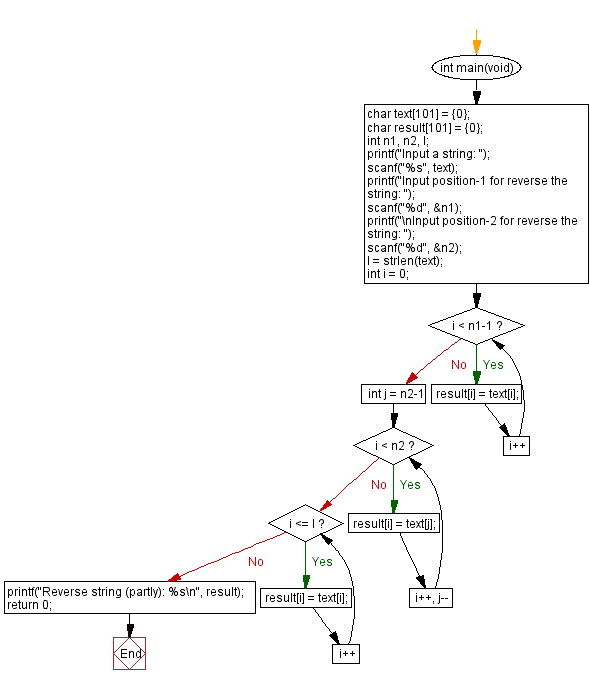﻿ C - Reverse a string partially# C Exercises: Reverse a string partially

## C Basic-II: Exercise-2 with Solution

Write a C program that takes a string and two integers (n1, n2). Now reverse the sequence of characters of the string between n1 and n2.
Let l be the length of the string.

Constraints:

• 1 ≤ n1 ≤ n2 ≤ l ≤100
• Each letter of the string is an uppercase or lowercase letter.

Sample Date:

("abcdxyabcd", 5, 6) -> "abcdyxabcd" ("Exercises", 1, 3) -> "exercises"

C Code:

``````#include <stdio.h>
#include <string.h>

int main(void)
{
char text = {0};
char result = {0};
int n1, n2, l;
printf("Input a string: ");
scanf("%s", text);
printf("Input position-1 for reverse the string: ");
scanf("%d", &n1);
printf("\nInput position-2 for reverse the string: ");
scanf("%d", &n2);

l = strlen(text);
int i = 0;
for(; i < n1-1; i++) {
result[i] = text[i];
}
for(int j = n2-1; i < n2; i++, j--) {
result[i] = text[j];
}
for(; i <= l; i++) {
result[i] = text[i];
}

printf("Reverse string (partly): %s\n", result);

return 0;
}
``````

Sample Output:

```Input a string: Input position-1 for reverse the string: abcdxyabcd 5 6
Input position-2 for reverse the string: Reverse string (partly): abcdyxabcd
```

Flowchart:C Programming Code Editor:

Contribute your code and comments through Disqus.

Previous C Programming Exercise: Find the integer that appears the least often.

What is the difficulty level of this exercise?

Test your Programming skills with w3resource's quiz.

﻿